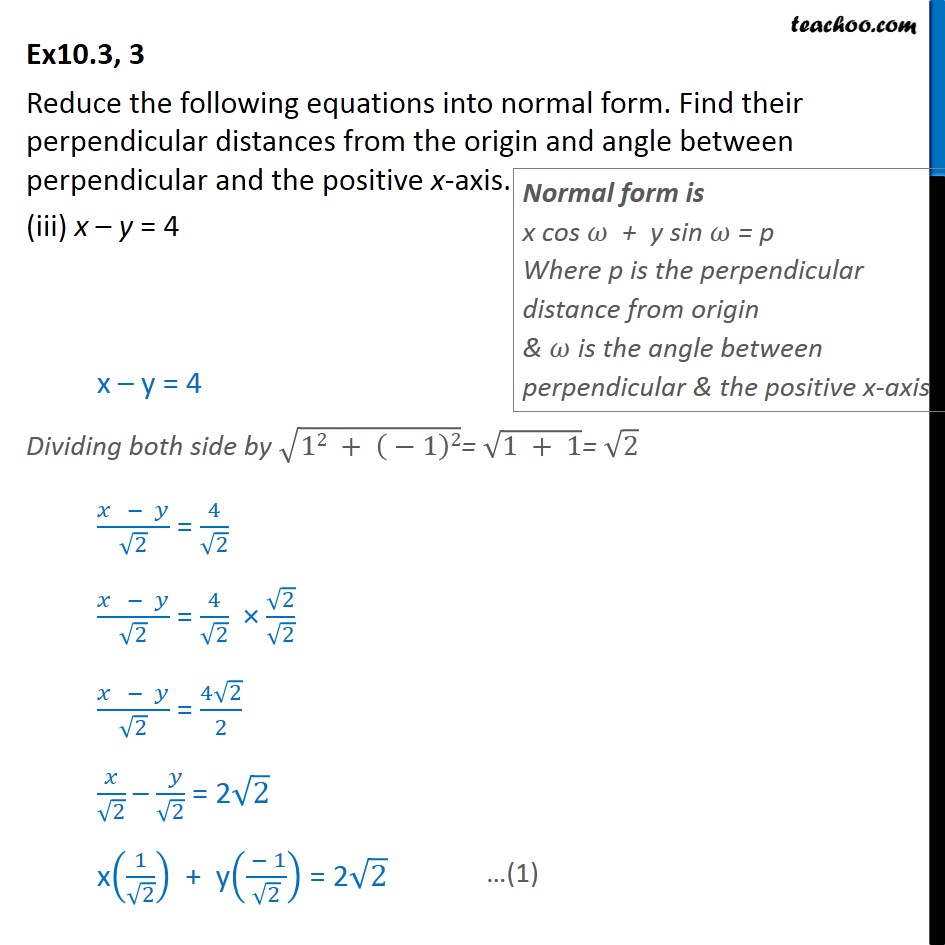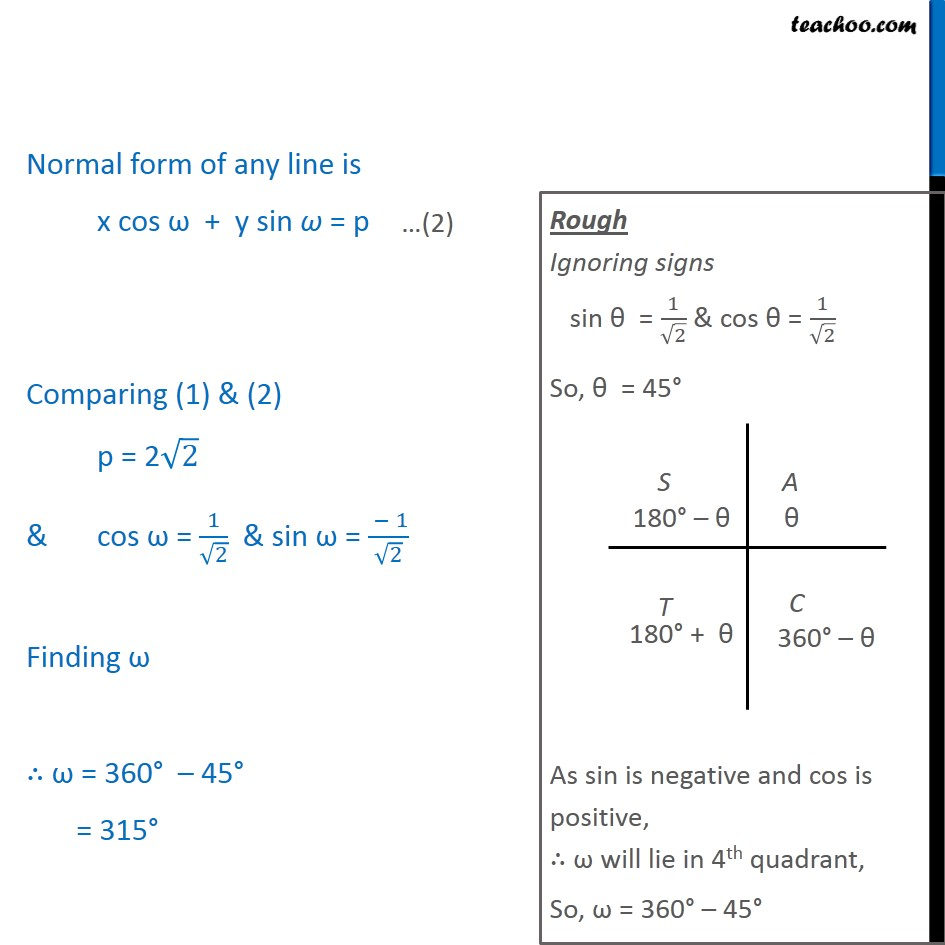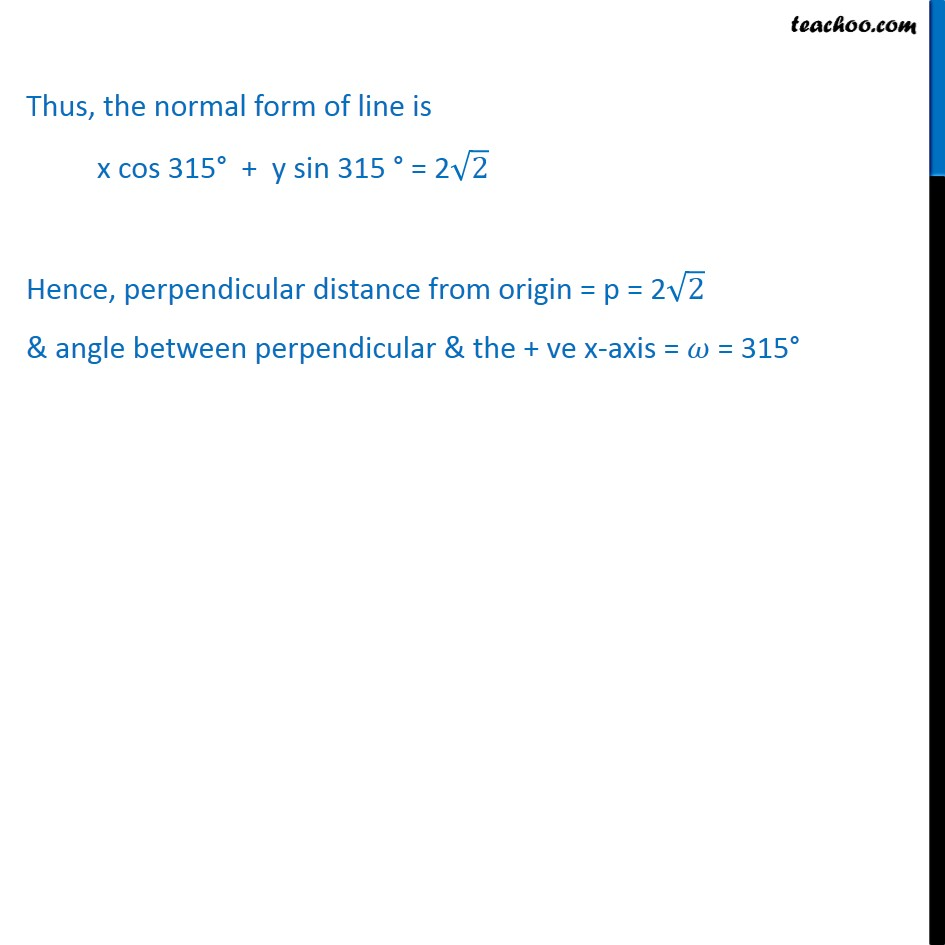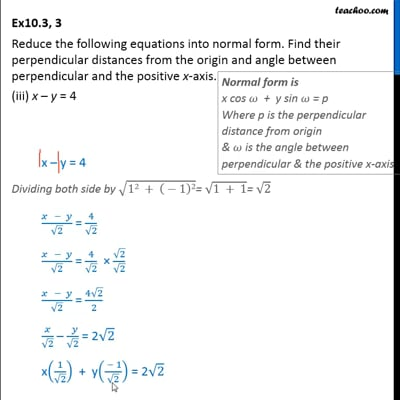Ex 10.3

Chapter 10 Class 11 Straight Lines
Serial order wiseThis video is only available for Teachoo black users

Introducing your new favourite teacher - Teachoo Black, at only ₹83 per month

### Transcript

Ex10.3, 3 Reduce the following equations into normal form. Find their perpendicular distances from the origin and angle between perpendicular and the positive x-axis. (iii) x y = 4 x y = 4 Dividing both side by (12 + ( 1)2)= (1 + 1)= 2 ( )/ 2 = 4/ 2 ( )/ 2 = 4/ 2 2/ 2 ( )/ 2 = (4 2)/2 / 2 ( )/ 2 = 2 2 x(1/ 2) + y(( 1)/ 2) = 2 2 Normal form of any line is x cos + y sin = p Comparing (1) & (2) p = 2 2 & cos = 1/ 2 & sin = ( 1)/ 2 Finding = 360 45 = 315 Thus, the normal form of line is x cos 315 + y sin 315 = 2 2 Hence, perpendicular distance from origin = p = 2 2 & angle between perpendicular & the + ve x-axis = = 315